• python实现棋盘覆盖问题及可视化
2021-04-27 08:15:39

问题介绍

棋盘覆盖问题，是一种编程问题。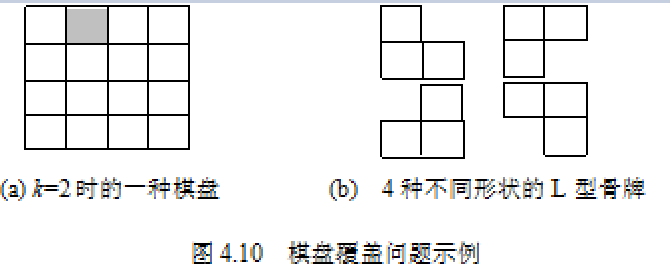如何应用分治法求解棋盘覆盖问题呢？分治的技巧在于如何划分棋盘，使划分后的子棋盘的大小相同，并且每个子棋盘均包含一个特殊方格，从而将原问题分解为规模较小的棋盘覆盖问题。k>0时，可将2k×2k的棋盘划分为4个2(k-1)×2(k-1)的子棋盘。这样划分后，由于原棋盘只有一个特殊方格，所以，这4个子棋盘中只有一个子棋盘包含该特殊方格，其余3个子棋盘中没有特殊方格。为了将这3个没有特殊方格的子棋盘转化为特殊棋盘，以便采用递归方法求解，可以用一个l型骨牌覆盖这3个较小棋盘的会合处，从而将原问题转化为4个较小规模的棋盘覆盖问题。递归地使用这种划分策略，直至将棋盘分割为1×1的子棋盘。

问题解释来源 百度

效果展示

k=1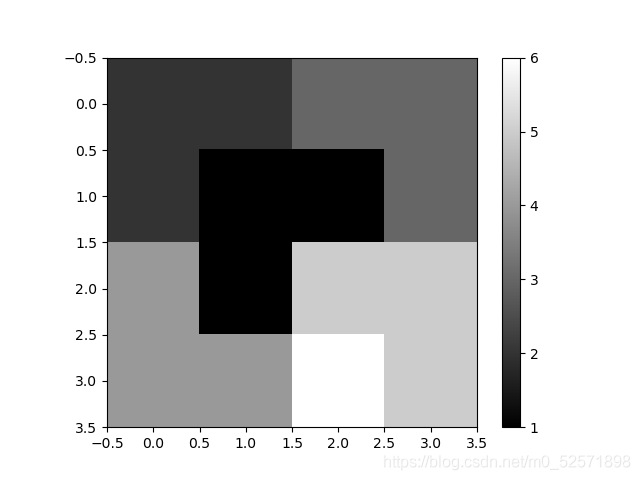k=2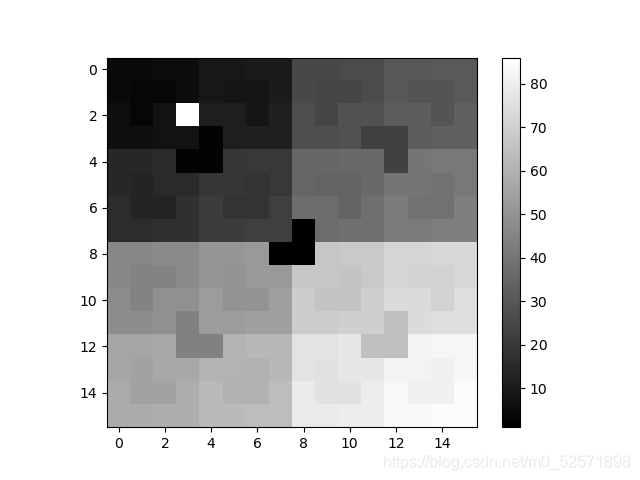代码实现

借助numpy处理数据，plot实现可视化。

使用面向对象的方法设计了棋盘类。

一步步将棋盘分为小区块，指导区块的边长为1，退出递归。

import numpy as np

import matplotlib.pyplot as plt

class board:

def __init__(self, size, x, y):

'''

初始化棋盘

:param size: 棋盘边长

:param x: 特殊点横坐标

:param y: 特殊点纵坐标

'''

self.special_block = (x, y)

self.board = np.zeros((size, size), dtype=int)

self.board[x][y] = (size * size - 1) / 3 + 1

self.t = 1

self.size = size

def visualize(self):

'''

可视化函数

:return: none

'''

plt.imshow(self.board, cmap=plt.cm.gray)

plt.colorbar()

plt.show()

def fill_block(self, x, y):

'''

填充点(x, y)

:param x: x

:param y: y

:return: none

'''

if self.board[x][y] == 0:

self.board[x][y] = self.t

else:

raise exception

def fill(self, s_x, s_y, size, c_x, c_y):

'''

递归函数填充棋盘或子棋盘(下文称区块)

:param s_x: 区块左上角x

:param s_y: 区块左上角y

:param size: 区块边长

:param c_x: 区块特殊点坐标x

:param c_y: 区块特殊点坐标x

:return: none

'''

if size == 1:

return

pos = (round((c_x - s_x + 1) / size), round((c_y - s_y + 1) / size))

center = (round(s_x + size / 2 - 1), round(s_y + size / 2 - 1))

ls = [(0, 0), (0, 1), (1, 0), (1, 1)] # 代表四个子区块

for i in ls:

if i != pos: # 如果不是原有特殊点所在区块，则构造特殊点并填充

x = center + i

y = center + i

self.fill_block(x, y)

self.t += 1 # 标记号加一，标记下一骨牌

for i in ls:

if i != pos: # 如果不是原有特殊点所在区块

# 所构造特殊点位置(x, y)

x = center + i

y = center + i

x1 = s_x + i * (size / 2)

y1 = s_y + i * (size / 2)

self.fill(x1, y1, size / 2, x, y)

else: # 如果是原有特殊点所在区块

x1 = s_x + i * (size / 2)

y1 = s_y + i * (size / 2)

self.fill(x1, y1, size / 2, c_x, c_y)

主函数

if __name__ == '__main__':

k = eval(input("请输入正整数k(棋盘大小2^2k,2^2k):\n"))

loc_x = eval(input("请输入特殊点横坐标:\n"))

loc_y = eval(input("请输入特殊点纵坐标:\n"))

size = 2 ** (2 * k)

b = board(size, loc_x, loc_y)

b.fill(0, 0, size, loc_x, loc_y)

b.visualize()

print(b.board)

总结

到此这篇关于python实现棋盘覆盖问题及可视化的文章就介绍到这了,更多相关python棋盘覆盖问题内容请搜索萬仟网以前的文章或继续浏览下面的相关文章希望大家以后多多支持萬仟网！

如您对本文有疑问或者有任何想说的，请点击进行留言回复，万千网友为您解惑！

更多相关内容
• 主要介绍了Python3解决棋盘覆盖问题的方法,简单描述了棋盘覆盖问题的概念、原理及Python相关操作技巧,需要的朋友可以参考下
• 主要介绍了Java基于分治算法实现的棋盘覆盖问题,简单描述了棋盘覆盖问题,并结合具体实例形式分析了java基于分治算法实现棋盘覆盖问题的相关操作技巧,需要的朋友可以参考下
• 贵州大学计算机科学与技术学院 计算机科学与技术系上机实验报告 课程...- 棋盘覆盖问题 二实验目的及要求 1熟悉递归算法编写 2理解分治算法的特点 3掌握分治算法的基本结构 三实验环境 Visual C++ 四实验内容 根据教材
• ## 棋盘覆盖问题

千次阅读 2022-03-12 12:28:30
棋盘覆盖三种实现方法详解

# 棋盘覆盖问题详解

1.问题描述：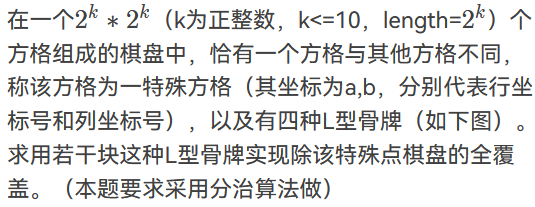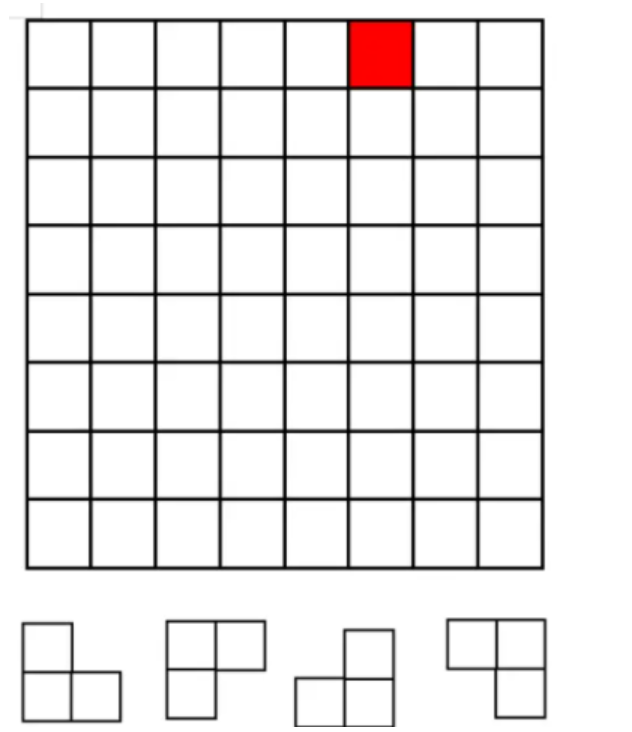## 方法一 分治法

• 首先回忆一下分治法的适用条件
• 1.问题规模缩小到一定程度后容易解决（当棋盘只有一个方格，则该方格必为特殊方格无需处理）。
• 2.问题能够被划分为若干个规模较小的相同子问题（我们考虑将大棋盘划分为四个大小相同的小棋盘，但是存在一个问题划分后只有一个小棋盘内含有特殊方格为和原问题相同的子问题，其余三个不含有特殊方格，不能使用分治方法解决。稍后解释~~~）。
• 3.子问题的解能够合并成为原问题的解（当小棋盘全部覆盖完毕，则原问题得到解决）。
• 4.不存在相同子问题（每次划分得到的小棋盘各不相同）。

解释2中的问题：
当k>0时，我们把规模为2k * 2k的大棋盘划分为2(k-1) * 2(k-1)的四个小棋盘。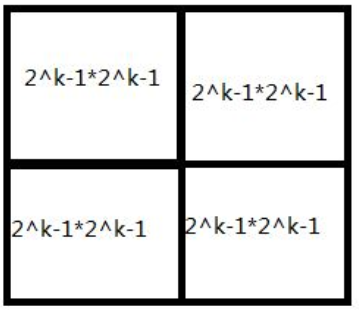则此时的特殊方格位于四个棋盘其中之一，为了将其余的三个棋盘也同样转化成和原问题相同的棋盘，我们只需要根据划分后特殊棋盘的位置选择合适的骨牌放置在大棋盘的汇合处。如图：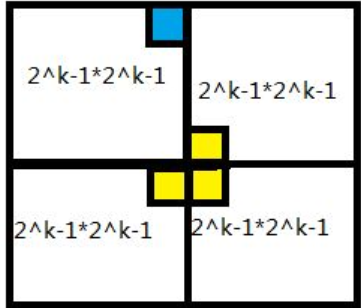这样就得到了四个和原问题相同的规模更小的子问题，再将子问题进行相同的处理，直到问题规模变为1直接返回。

代码分析

#include<bits/stdc++.h>
#include<windows.h>
using namespace std;
const int N=1100;
int g[N][N]; //棋盘数组
int title=1; //骨牌编号
void SetColor(int fore = 7, int back = 0)
{
SetConsoleTextAttribute(GetStdHandle(STD_OUTPUT_HANDLE), (back << 4) + fore);
}//背景颜色
void solve(int tr,int tc,int dr,int dc,int size){
/*tr棋盘左上角行号，tc棋盘左上角列号，size棋盘宽度，dr特殊位置行号，dc特殊位置列号*/
if(size==1) return; //递归出口
int t=title++;
int s=size/2; //分割棋盘
//处理左上角部分
if(dr<tr+s&&dc<tc+s){
solve(tr,tc,dr,dc,s);
}else{
g[tr+s-1][tc+s-1]=t;
solve(tr,tc,tr+s-1,tc+s-1,s);
}
//处理右上角部分
if(dr<tr+s&&dc>=tc+s){
solve(tr,tc+s,dr,dc,s);
}else{
g[tr+s-1][tc+s]=t;
solve(tr,tc+s,tr+s-1,tc+s,s);
}
//处理右下角部分
if(dr>=tr+s&&dc>=tc+s){
solve(tr+s,tc+s,dr,dc,s);
}else{
g[tr+s][tc+s]=t;
solve(tr+s,tc+s,tr+s,tc+s,s);
}
//处理左下角部分
if(dr>=tr+s&&dc<tc+s){
solve(tr+s,tc,dr,dc,s);
}else{
g[tr+s][tc+s-1]=t;
solve(tr+s,tc,tr+s,tc+s-1,s);
}
}
int main(){
int a,b,legth;
cin>>a>>b>>legth;
solve(1,1,a,b,legth);
for(int i=1;i<=legth;i++){
for(int j=1;j<=legth;j++){
if(g[i][j]){
SetColor(0, g[i][j]);
printf("%4d",g[i][j]);
}
else{
printf("%4d",g[i][j]);
}
}
printf("\n");
}
return 0;
}


代码思路：
输入棋盘左上角的坐标（此处为(1,1)）和棋盘的宽度，然后借助solve函数进行处理，每次将原棋盘分割为4个小棋盘，然后根据左上、右上、右下、左下的顺时针顺序进行处理，如果特殊方格存在于该小棋盘则直接递归处理该小棋盘。否则，先将该小棋盘的指定位置覆盖上骨牌转换成和原问题相同的问题后在进行分割处理。

## 方法二 非递归化

### 借助队列实现

实现思路
借助结构体存储棋盘相关信息（左上角的坐标，特殊方格的位置坐标，棋盘的宽度），先将原棋盘入队列，每次取出队首的元素，当队首元素的规模不为1时，对其进行处理，再将分割后的子棋盘入队，不断循环直至队列为空。

#include<bits/stdc++.h>
#include<windows.h>
void SetColor(int fore = 7, int back = 0)
{
SetConsoleTextAttribute(GetStdHandle(STD_OUTPUT_HANDLE), (back << 4) + fore);
}//背景颜色
using namespace std;
const int N=1100;
int g[N][N];
int title=1;
struct Point{
int x;
int y;
int tx;
int ty;
int size;
}p;
queue<Point> q;
int main(){
int a,b,length;
cin>>a>>b>>length;
q.push({1,1,a,b,length});
while(!q.empty()){
Point c=q.front(); //取出队首元素
int tr,tc,dr,dc;
if(c.size!=1){
int s=c.size/2;
int t=title++;
tr=c.x;
tc=c.y;
dr=c.tx;
dc=c.ty;
if(dr<tr+s&&dc<tc+s){
q.push({tr,tc,dr,dc,s});
}else{
g[tr+s-1][tc+s-1]=t;
q.push({tr,tc,tr+s-1,tc+s-1,s});
}

if(dr<tr+s&&dc>=tc+s){
q.push({tr,tc+s,dr,dc,s});
}else{
g[tr+s-1][tc+s]=t;
q.push({tr,tc+s,tr+s-1,tc+s,s});
}

if(dr>=tr+s&&dc>=tc+s){
q.push({tr+s,tc+s,dr,dc,s});
}else{
g[tr+s][tc+s]=t;
q.push({tr+s,tc+s,tr+s,tc+s,s});
}

if(dr>=tr+s&&dc<tc+s){
q.push({tr+s,tc,dr,dc,s});
}else{
g[tr+s][tc+s-1]=t;
q.push({tr+s,tc,tr+s,tc+s-1,s});
}
q.pop();
}else{
//q.pop();
break;
}
}

for(int i=1;i<=length;i++){
for(int j=1;j<=length;j++){
if(g[i][j]){
SetColor(0, g[i][j]);
printf("%4d",g[i][j]);
}
else{
printf("%4d",g[i][j]);
}
}
printf("\n");
}
return 0;
}


注： 在处理时发现，只要此时队首元素的规模为1，则队列中其余元素的规模全为1都无需进行处理，因此当出现队首元素为1时可以直接终止循环，无需等到队列为空。

### 借助栈实现

实现思路
借助结构体存储棋盘相关信息（左上角的坐标，特殊方格的位置坐标，棋盘的宽度），先将原棋盘入栈，每次取出栈顶的元素，当栈顶元素的规模不为1时，对其进行处理，再将分割后的子棋盘入栈，不断循环直至栈为空。

#include<bits/stdc++.h>
#include<windows.h>
using namespace std;
const int N=1100;
int g[N][N];
int title=1;
void SetColor(int fore = 7, int back = 0)
{
SetConsoleTextAttribute(GetStdHandle(STD_OUTPUT_HANDLE), (back << 4) + fore);
}//背景颜色
struct Point{
int x;
int y;
int tx;
int ty;
int size;
}p;
stack<Point> st;
int main(){
int a,b,length;
cin>>a>>b>>length;
st.push({1,1,a,b,length});

while(!st.empty()){
Point c=st.top();
int tr,tc,dr,dc;
if(c.size!=1){
int s=c.size/2;
int t=title++;
tr=c.x;
tc=c.y;
dr=c.tx;
dc=c.ty;
st.pop();
if(dr<tr+s&&dc<tc+s){
st.push({tr,tc,dr,dc,s});
}else{
g[tr+s-1][tc+s-1]=t;
st.push({tr,tc,tr+s-1,tc+s-1,s});
}

if(dr<tr+s&&dc>=tc+s){
st.push({tr,tc+s,dr,dc,s});
}else{
g[tr+s-1][tc+s]=t;
st.push({tr,tc+s,tr+s-1,tc+s,s});
}

if(dr>=tr+s&&dc>=tc+s){
st.push({tr+s,tc+s,dr,dc,s});
}else{
g[tr+s][tc+s]=t;
st.push({tr+s,tc+s,tr+s,tc+s,s});
}

if(dr>=tr+s&&dc<tc+s){
st.push({tr+s,tc,dr,dc,s});
}else{
g[tr+s][tc+s-1]=t;
st.push({tr+s,tc,tr+s,tc+s-1,s});
}
}else{
st.pop();
//break;
}
}

for(int i=1;i<=length;i++){
for(int j=1;j<=length;j++){
if(g[i][j]){
SetColor(0, g[i][j]);
printf("%4d",g[i][j]);
}
else{
printf("%4d",g[i][j]);
}
}
printf("\n");
}
return 0;
}


附加
为了帮助大家清晰的理解棋盘覆盖，三种实现方法的具体步骤，笔者采用python编写了可视化的小工具。
棋盘覆盖工具.

展开全文c++ 算法 开发语言
• 实验报告 学号 课程 0107 算法分析与设计 姓名 高行行 实验日期 专业班级 移动互联网实验时间 14-01 8:00-9:00 实验情况 备注 棋盘覆盖问题算法 #include> int tile=1; int board; void ChessBoard(int tr...
• 在一个2^k*2^k个方格组成的棋盘中，恰有一个方格与其他方格不同...棋盘覆盖问题要求下图四种不同形状的L型骨牌覆盖给定棋盘上除特殊方格以外的所有方格，且任意2个L型骨牌不得重叠覆盖。 （仅供参考，请独立完成实验）
• 分治法实现棋盘的“3-L形”完全覆盖，java实现。
• 在一个2k*2k个方格组成的棋盘中，若恰有一个方格与其他方格不同，则称该方格位一...在棋盘覆盖问题中，要用4种不同形态的L型骨牌覆盖一个给定的特殊棋盘上除特殊方格以外的所有方格，且任何2个L型骨牌不得重叠覆盖。
• 课程的随堂作业，C语言的，用dev就能运行，萌新代码，勿喷，仅仅帮助不想写作业的朋友方便一下，反正老师也不会仔细检查的
• python怎么实现棋盘覆盖问题及可视化发布时间：2021-03-12 17:04:06来源：亿速云阅读：94作者：TREX这篇文章主要介绍“python怎么实现棋盘覆盖问题及可视化”，在日常操作中，相信很多人在python怎么实现棋盘覆盖...

python怎么实现棋盘覆盖问题及可视化

发布时间：2021-03-12 17:04:06

来源：亿速云

阅读：94

作者：TREX

这篇文章主要介绍“python怎么实现棋盘覆盖问题及可视化”，在日常操作中，相信很多人在python怎么实现棋盘覆盖问题及可视化问题上存在疑惑，小编查阅了各式资料，整理出简单好用的操作方法，希望对大家解答”python怎么实现棋盘覆盖问题及可视化”的疑惑有所帮助！接下来，请跟着小编一起来学习吧！

问题介绍

棋盘覆盖问题，是一种编程问题。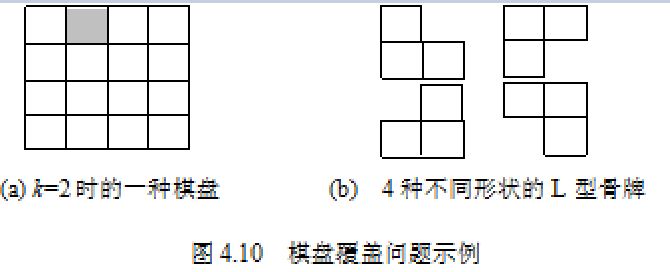如何应用分治法求解棋盘覆盖问题呢？分治的技巧在于如何划分棋盘，使划分后的子棋盘的大小相同，并且每个子棋盘均包含一个特殊方格，从而将原问题分解为规模较小的棋盘覆盖问题。k>0时，可将2k×2k的棋盘划分为4个2(k-1)×2(k-1)的子棋盘。这样划分后，由于原棋盘只有一个特殊方格，所以，这4个子棋盘中只有一个子棋盘包含该特殊方格，其余3个子棋盘中没有特殊方格。为了将这3个没有特殊方格的子棋盘转化为特殊棋盘，以便采用递归方法求解，可以用一个L型骨牌覆盖这3个较小棋盘的会合处，从而将原问题转化为4个较小规模的棋盘覆盖问题。递归地使用这种划分策略，直至将棋盘分割为1×1的子棋盘。

问题解释来源 百度

原网页

效果展示

k=1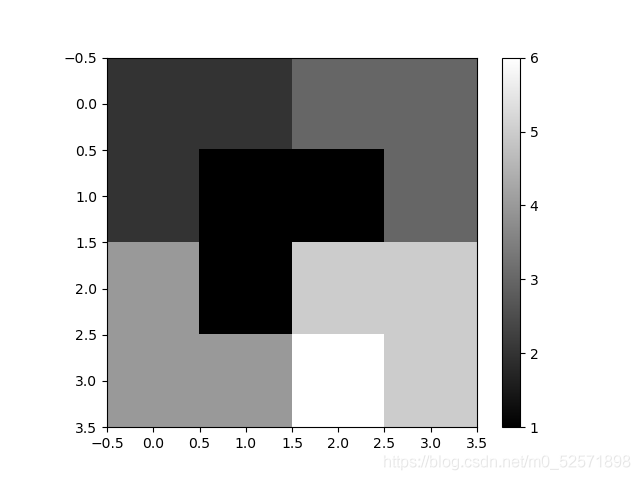k=2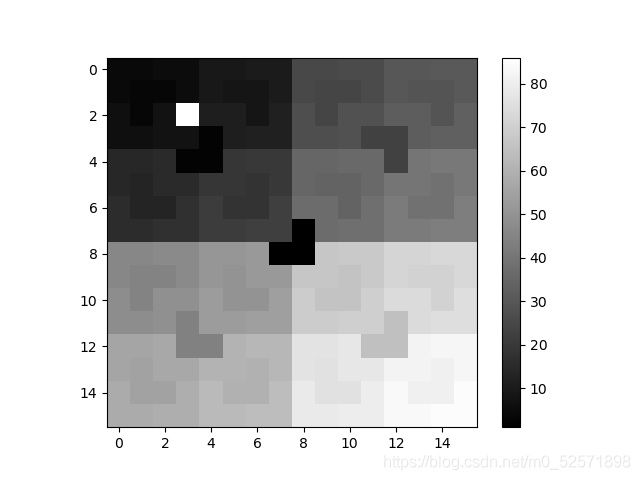代码实现

借助numpy处理数据，plot实现可视化。

使用面向对象的方法设计了棋盘类。

一步步将棋盘分为小区块，指导区块的边长为1，退出递归。import numpy as np

import matplotlib.pyplot as plt

class Board:

def __init__(self, size, x, y):

'''

初始化棋盘

:param size: 棋盘边长

:param x: 特殊点横坐标

:param y: 特殊点纵坐标

'''

self.special_block = (x, y)

self.board = np.zeros((size, size), dtype=int)

self.board[x][y] = (size * size - 1) / 3 + 1

self.t = 1

self.size = size

def visualize(self):

'''

可视化函数

:return: None

'''

plt.imshow(self.board, cmap=plt.cm.gray)

plt.colorbar()

plt.show()

def fill_block(self, x, y):

'''

填充点(x, y)

:param x: x

:param y: y

:return: None

'''

if self.board[x][y] == 0:

self.board[x][y] = self.t

else:

raise Exception

def fill(self, s_x, s_y, size, c_x, c_y):

'''

递归函数填充棋盘或子棋盘(下文称区块)

:param s_x: 区块左上角x

:param s_y: 区块左上角y

:param size: 区块边长

:param c_x: 区块特殊点坐标x

:param c_y: 区块特殊点坐标x

:return: None

'''

if size == 1:

return

pos = (round((c_x - s_x + 1) / size), round((c_y - s_y + 1) / size))

center = (round(s_x + size / 2 - 1), round(s_y + size / 2 - 1))

ls = [(0, 0), (0, 1), (1, 0), (1, 1)] # 代表四个子区块

for i in ls:

if i != pos: # 如果不是原有特殊点所在区块，则构造特殊点并填充

x = center + i

y = center + i

self.fill_block(x, y)

self.t += 1 # 标记号加一，标记下一骨牌

for i in ls:

if i != pos: # 如果不是原有特殊点所在区块

# 所构造特殊点位置(x, y)

x = center + i

y = center + i

x1 = s_x + i * (size / 2)

y1 = s_y + i * (size / 2)

self.fill(x1, y1, size / 2, x, y)

else: # 如果是原有特殊点所在区块

x1 = s_x + i * (size / 2)

y1 = s_y + i * (size / 2)

self.fill(x1, y1, size / 2, c_x, c_y)

主函数if __name__ == '__main__':

k = eval(input("请输入正整数K(棋盘大小2^2k,2^2k):\n"))

loc_x = eval(input("请输入特殊点横坐标:\n"))

loc_y = eval(input("请输入特殊点纵坐标:\n"))

size = 2 ** (2 * k)

b = Board(size, loc_x, loc_y)

b.fill(0, 0, size, loc_x, loc_y)

b.visualize()

print(b.board)

GitHub链接

总结

到此，关于“python怎么实现棋盘覆盖问题及可视化”的学习就结束了，希望能够解决大家的疑惑。理论与实践的搭配能更好的帮助大家学习，快去试试吧！若想继续学习更多相关知识，请继续关注亿速云网站，小编会继续努力为大家带来更多实用的文章！

展开全文• 这是我们校选课上的一个题目，利用分治算法去解棋盘覆盖问题算是最简单的办法吧。在还没加入校队前就看到过这个题目，当时真的有种没法入手，也许那时真的什么都不懂吧，根本也没想过到底怎么入手。自从加入校队，...
• 当四等分后为2*2的棋盘且特殊方格在其中，则刚好用一个L填满棋盘。 解题思路：先将棋盘按中间点四等...//棋盘覆盖问题 public class ChessBoardCoverage { private static int BOARD_SIZE = 4;//例举4*4的棋盘 pr...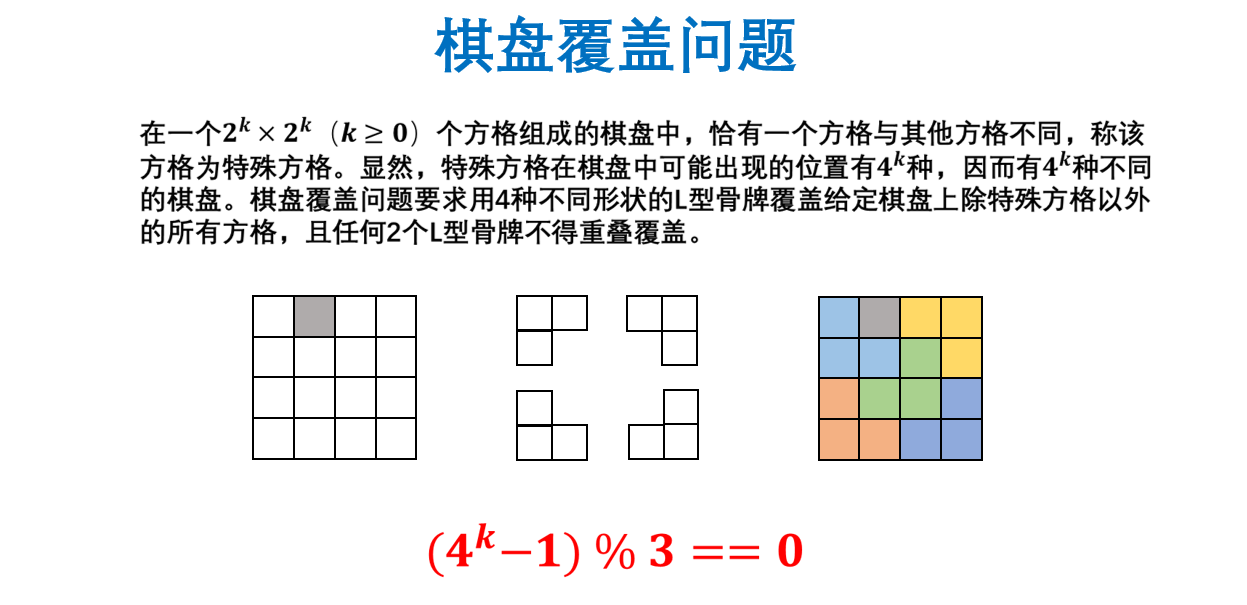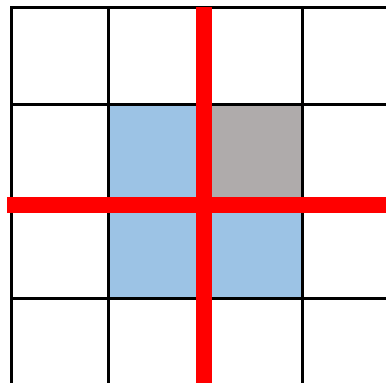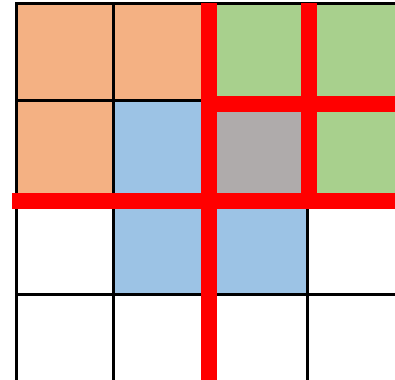当四等分后为2*2的棋盘且特殊方格在其中，则刚好用一个L填满棋盘。

解题思路：先将棋盘按中间点四等分为左上，右上，左下，右下四部分。
**当四等分后为22的棋盘且特殊方格在其中，则刚好用一个L填满棋盘。
若四等分后特殊方格不在其中，则将中心四格除了特殊方格在的部分其他三个格子涂同一颜色（如特殊方格在右上部分，则将左上、左下、右下涂色），并标记为特殊方格。然后再将每个部分四等分，若每部分大于2
2则继续标记，直到等分后为2*2的棋盘，用一个L填满棋盘。

//棋盘覆盖问题
public class ChessBoardCoverage {
private static int BOARD_SIZE = 8;//例举8*8的棋盘
private static int[][] board = new int[BOARD_SIZE][BOARD_SIZE];
private static int title = 0;

public static void main(String[] args) {
Scanner input = new Scanner(System.in);
System.out.println(">输入特殊方格的角标：");
int dr = input.nextInt();
int dc = input.nextInt(); //dr，dc为特殊点的下标
//tr，tc为基准点，用于标记每个部分并进行操作
chessBoard(0, 0, dr, dc, BOARD_SIZE);
printBoard();
}
//输出二维数组内容
private static void printBoard() {
for (int i = 0;i < BOARD_SIZE;i++){
for (int j = 0;j < BOARD_SIZE;j++){
System.out.print(board[i][j] + "\t");
}
System.out.println();
}
}
//chessBoard（基准点行下标，基准点列下标，特殊点行下标，特殊点列下标，当前部分是size*size）
private static void chessBoard(int tr, int tc, int dr, int dc, int size) {
if (size == 1) {
return;//返回上一层，从哪里调用chessBoard进来的就返回哪里
}
int num = ++title; //因为title是static型，所以在返回上一层时num是当前层的值不变，但进入新的一层时都会是上一次递进时的num+1且不变
int s = size / 2;
//左上 四个方向在同一层是并列关系
if (dr < tr + s && dc < tc + s) {
chessBoard(tr,tc,dr,dc,s);
} else {
board[tr + s - 1][tc + s - 1] = num;
chessBoard(tr,tc,tr + s - 1,tc + s - 1,s);
}

//右上
if (dr < tr + s && dc >= tc + s) {
chessBoard(tr,tc + s,dr,dc,s);
} else {
board[tr + s - 1][tc + s] = num;
chessBoard(tr,tc + s,tr + s - 1,tc + s,s);
}

//左下
if (dr >= tr + s && dc < tc + s) {
chessBoard(tr + s,tc,dr,dc,s);
} else {
board[tr + s][tc + s - 1] = num;
chessBoard(tr + s,tc,tr + s,tc + s - 1,s);
}

//右下
if (dr >= tr + s && dc >= tc + s) {
chessBoard(tr + s,tc + s,dr,dc,s);
} else {
board[tr + s][tc + s] = num;
chessBoard(tr + s,tc + s,tr + s,tc + s,s);
}
}
}


测试结果：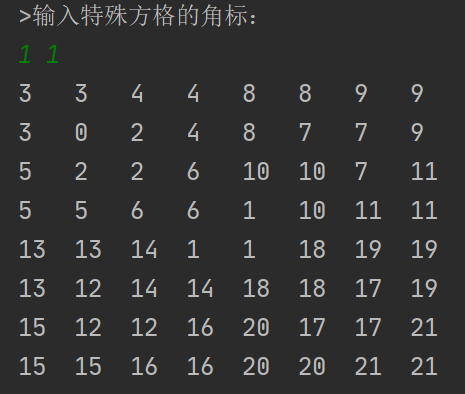展开全文java 开发语言 后端
•java 算法...